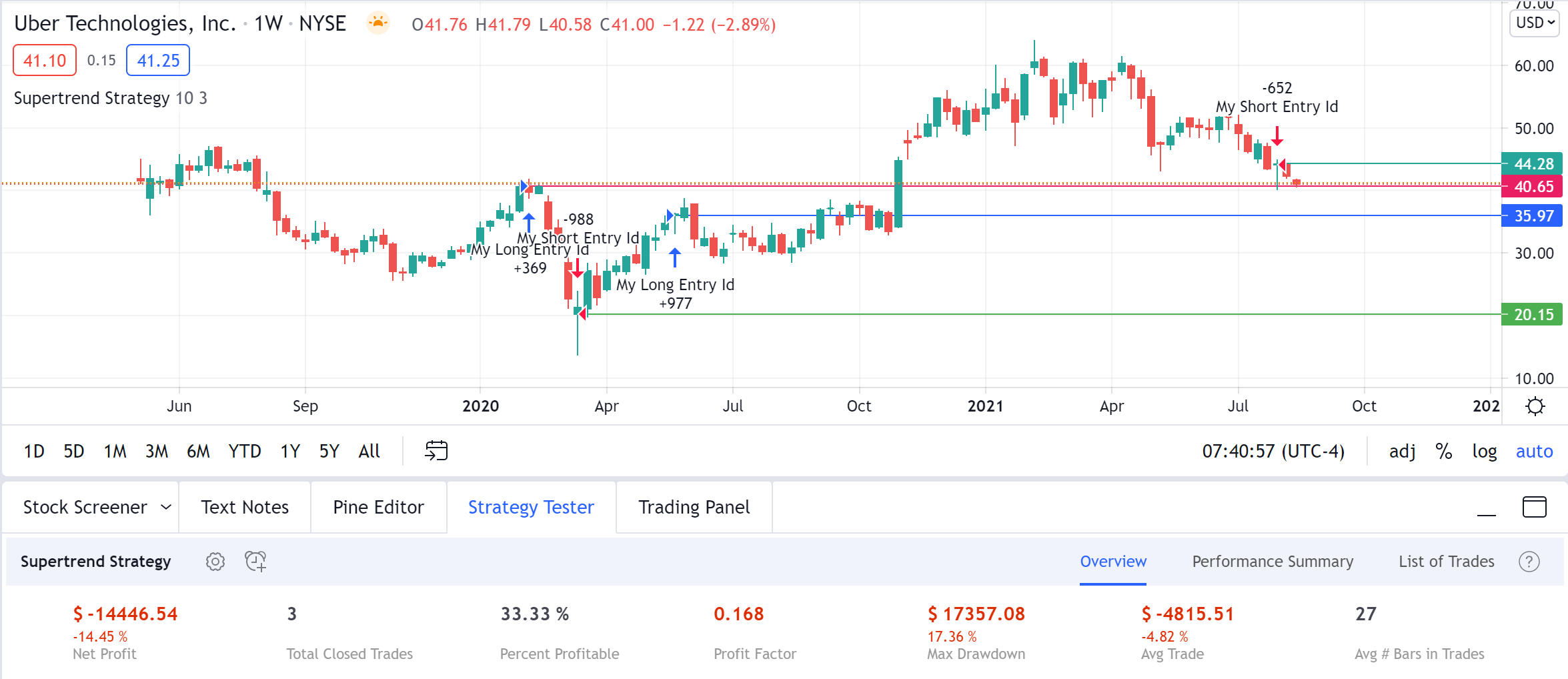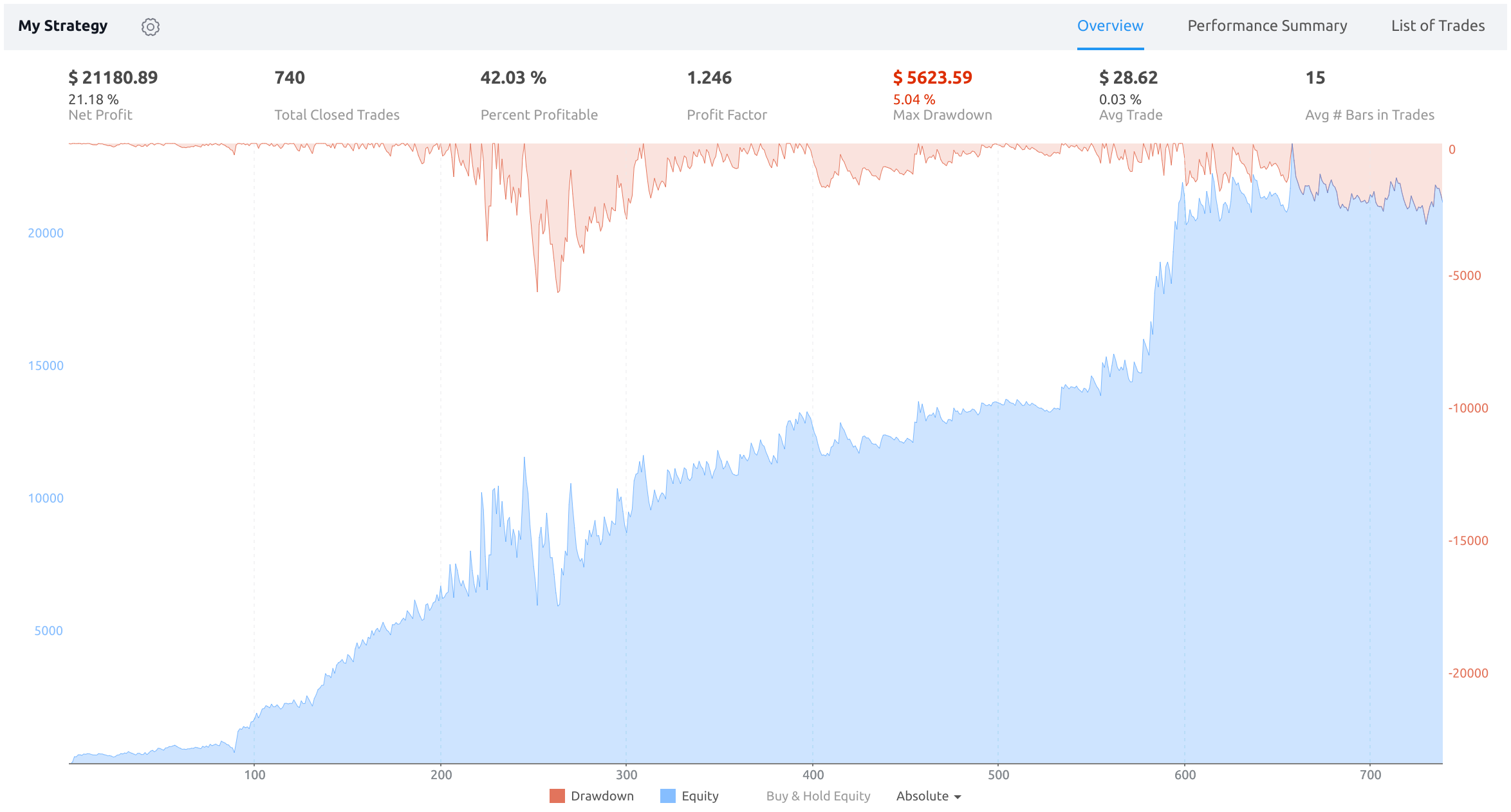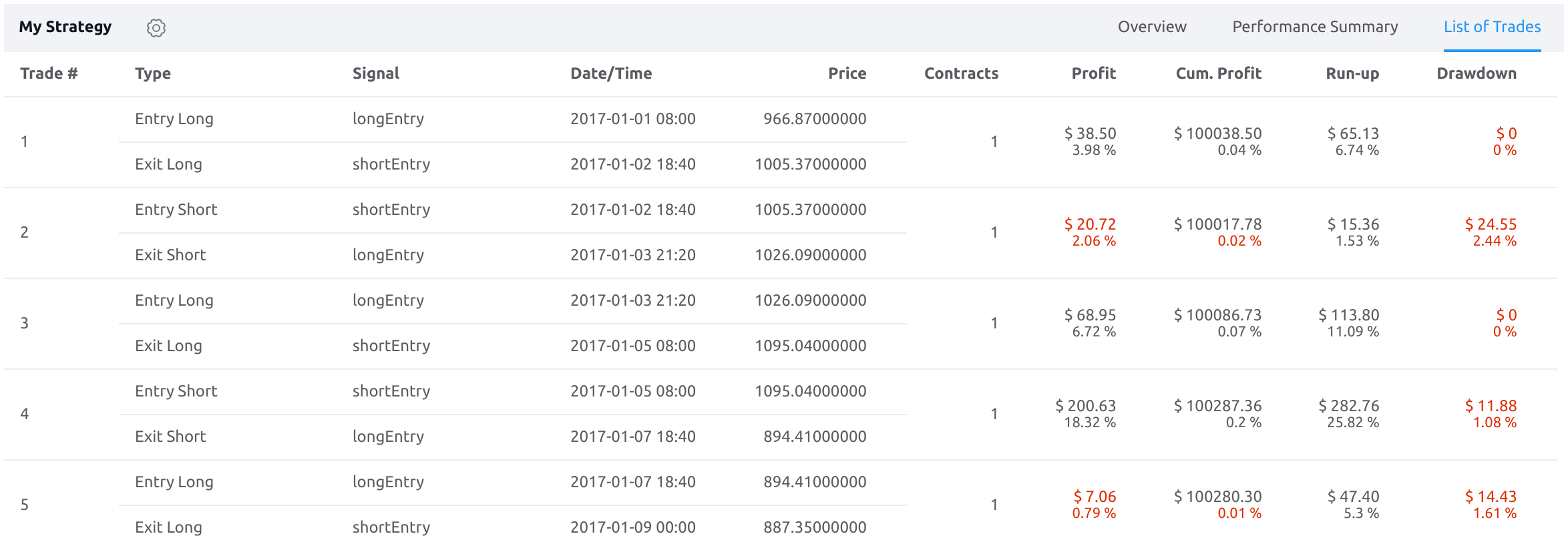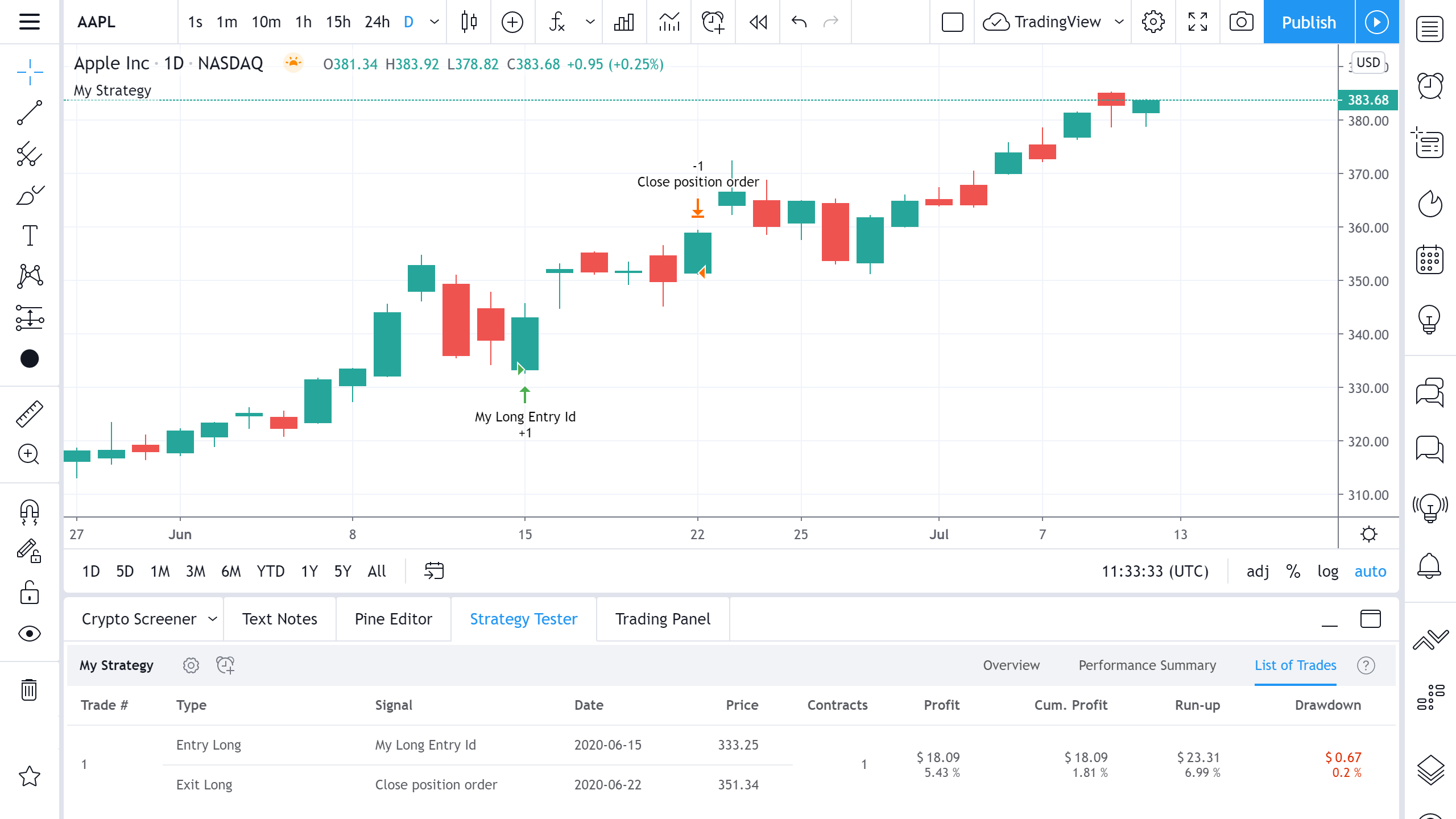# How are Strategy Tester Report values calculated and what do they mean?

#### Performance Summary Tab

This tab displays all available performance metrics for the strategy including Net Profit, Gross Profit, Max Drawdown and more. It looks like this:The performance metrics calculated for all trades are shown in the All column. Values calculated only for long and short trades are shown in the Long and Short columns, respectively. Now let’s dive into what each performance metric means.

Net Profit
The overall profit or loss (in the selected currency) achieved by the trading strategy in the test period. The value is the sum of all values from the Profit column (on the List of Trades tab), taking into account the sign.

Gross Profit
The total profit for all profitable trades generated by a strategy.

Gross Loss
The total losses for all losing trades generated by a strategy. Analyzing and reducing trade losses is an extremely important part of trading strategy analysis. That’s why this characteristic of a strategy is the most important. It should be noted that net profit increases not only when gross profit improves, but also when gross loss is reduced.

Max Drawdown
Displays the greatest loss drawdown, i.e. the greatest possible loss the strategy had during its run compared to its highest profits. To calculate the Max Drawdown that is displayed in the Overview tab in the Strategy Tester, we:

1. Calculate resulting Equity after each trade that the strategy closes, at the bar where it closed.

2. For each separate trade, calculate Max Equity at the time the trade closed. To do so, we take the strategy's Initial Capital and all of the Equity values from the trades that were already closed at that point and find the largest number among these values.

3. For each trade, calculate its own Drawdown. This is done by taking the trade's Max Equity (found in Step 2) and subtracting its current Equity (Step 1).

4. Take individual Drawdowns of all trades (Step 3) and find the largest number among them.

Max Drawdown is the hardest the strategy falls, equity-wise, from its peaks. You can simplify its formula as "Highest Equity of all time - Lowest Equity after the highest peak". You can roughly see this value on an equity curve graph by looking from the highest peaks to the lowest lows moving forward. Let's look at how Max Drawdown is calculated:Starting Equity (i.e. the funds available to the strategy) is always equal to Initial Capital. In our case, it is 100000.

On our first trade, the strategy enters long and buys 369 contracts for 40.65 = buys 14 999.85 USD worth of stock.

On our second trade, the strategy gets a signal to reverse its position. To do so, we would have to sell our 369 long contracts (to get to 0 position size) and then sell some more to have a short position. We sell 369 contracts at 20.15 = get 7 435.35 USD. Our equity after this trade is 100000 - 14 999,85 + 7 435,35 = 92435.5 USD. Our new Max Drawdown 100000 - 92435.5 = 7564.5 (it is automatically Max Drawdown because it is the only Drawdown value we have). After getting to 0, we also short 988-369=619 stocks at 20.15, effectively gaining 12472.85 USD (we short the stock, so we loan it and sell it expecting to buy it back later at a better price).

On our third trade, we get the signal to flip to long. To do that, we first need to buy back 619 stocks to close our short. The price went up, so it costs us 619 * 35.97 = 22265.43. To cover our position, we've spent 22265.43 and only earned 12472.85, so our loss for this trade is 9792.58. Our equity at this step is 92435.5-9792.58 = 82642.92. This is the lowest point our equity has been at, so our new Max Drawdown is 100000 - 82642.92 = 17357.08.

After that, we go long at 35.97 and then turn the position at around 44.28. The precise numbers are not important for us because this trade is actually profitable, thus it increases Equity. Max Drawdown still stays equal to 17357.08 because it is calculated based on peaks and troughs of Equity, and on this particular trade, our Equity is not at all time low.

The percentage and absolute values of a drawdown are two different metrics. They are tracked independently. For example, let’s say the initial capital is \$100. After a series of losing trades, equity decreases to \$50. The drawdown amounts to \$50 in absolute terms and 50% in relative terms. Later, after a series of profitable trades, equity increases to \$300 and then drops to \$200. In this case, the absolute drawdown will be \$100, and the relative drawdown, 33%. The overall maximum absolute drawdown of the strategy will be \$100, and the maximum relative drawdown will be 50%.

Note that drawdowns in the List of Trades are calculated differently. In List of Trades, Drawdown is recalculated on each bar (so each trades drawdown represents maximum possible loss during the trade). In Overview, Max Drawdown is recalculated not on each bar but on each closed trade.

The return achieved if all funds (Initial Capital) were used to buy the security when the first trade is entered, and the position was held for the duration of the test period.

Sharpe Ratio
Nobel Laureate, William Sharpe, introduced the Sharpe Ratio in 1966 under the name “reward-to-variability ratio”. The Sharpe Ratio is widely used by portfolio managers and individual traders to show how much risk was taken to achieve specific returns. The formula for the Sharpe ratio is SR = (MR - RFR) / SD, where MR is the average return for a period (monthly for a trading period of 3 or more months or daily for a trading period of 3 or more days), and RFR is the risk-free rate of return (by default, 2% annually). SD is the standard deviation of returns. Thus, this formula yields a value that could be loosely defined as return per unit risked if we accept the premise that variability is risk. The higher Sharpe ratio, the smoother the equity curve. Having a smooth equity curve is an important objective for many traders.

Sortino Ratio
The Sortino ratio is a variation of the Sharpe ratio. Unlike the Sharpe ratio, its calculation is done using the standard deviation of the downside risk, rather than that of the entire (upside + downside) risk. Due to this, it is thought to give a better view of a portfolio's risk-adjusted performance because positive volatility is considered a benefit.

Profit Factor
The amount of money a trading strategy made for every unit of money it lost (in the selected currency). This value is calculated by dividing gross profits by gross losses.

Max Contracts Held
The maximum number of contracts held at any one time.

Open PL
The profit or loss for the current open position. If no position is open, the value returned is N/A.

Commission Paid
The sum (in the selected currency) of commission paid. Slippage is not included.

The total number of closed trades (both winning and losing) generated by a strategy. The total number of trades is important for a number of reasons. First, the number should be large enough for strategy results to be of any statistical significance. Second, the number can help validate that your strategy is trading at the frequency you expect.

The number of entries currently opened.

The total number of winning trades generated by a strategy.

The total number of losing trades generated by a strategy.

Percent Profitable
The percentage of winning trades generated by a strategy. Calculated by dividing the number of winning trades by the total number of closed trades generated by a strategy. Percent profitable is not a very reliable measure by itself. A strategy could have many small winning trades, making the percent profitable high with a small average winning trade, or a few big winning trades accounting for a low percent profitable and a big average winning trade. Some successful strategies have a percent profitability below 50% but are still profitable due to proper loss control.

The sum of money gained or lost by the average trade generated by a strategy. Calculated by dividing the Net Profit by the overall number of closed trades. An important value since it must be large enough to cover the commission and slippage costs of trading the strategy and still bring a profit.

The Gross Profit divided by the number of Winning Trades generated by a strategy.

The Gross Loss divided by the number of Losing Trades generated by a strategy.

Ratio Avg Win / Avg Loss
The average value of how many currency units you win for every unit you lose (in the selected currency). This is calculated by dividing the average winning trade by the average losing trade. This field is not a very meaningful value by itself because it does not take into account the ratio of the number of winning vs losing trades, and strategies can have different approaches to profitability. A strategy may trade at every possibility in order to capture many small profits, yet have an average losing trade greater than the average winning trade. The higher this value is, the better, but it should be considered together with the percentage of winning trades and the net profit.

The most profitable trade in the test period.

The most losing trade in the test period.

The average number of bars that elapsed during trades for all closed trades.

Avg # Bars in Winning Trades
The average number of bars that elapsed during trades for all winning trades.

Avg # Bars in Losing Trades
The average number of bars that elapsed during trades for all losing trades.

Margin Calls
The total number of margin calls generated by a strategy.

#### Overview tab

This tab provides key performance metrics for the strategy.At the top of the tab are the metrics of the same name from the Performance Summary tab. The following charts are located in the center:

Drawdown

Equity
This chart displays the equity (in the selected currency) vs. trade number for all closed trades. The equity curve line chart presents trading performance on a trade-by-trade basis. This all-purpose equity chart is best used for general analysis of trading performance.

B&H
This chart displays the return achieved if all funds (Initial Capital) were used to buy the security when the first trade is entered, and the position was held for the duration of the test period. You can hide/show chart elements by clicking on their corresponding button at the bottom of the tab.

This tab displays detailed information on each trade.A trade is a pair of orders: an entry order and an exit order. Trades are arranged chronologically by execution of entry orders. The earliest trade is at the very top of the list.

Contains the sequential number of the trade.

Type
The direction of the trade (long or short).

Signal
The identifier of the order used to open or close the trade. An identifier is a string value assigned to the id argument of one of the following functions: strategy.entry, strategy.order, strategy.exit, strategy.close and strategy.close_all.

Date/Time
The transaction time in the chart's time zone.

Price
The execution price.

Contracts
The number of units bought or sold.

Profit

Cumulative Profit
The cumulative profits or losses of the strategy after closing the trade and the gain/loss percentage of the strategy over time.

Run-up
The maximum possible profit of the trade according to the strategy, as well as the maximum percentage gain.

Drawdown
The maximum possible loss of the trade according to the strategy, as well as the maximum percentage loss.

Let's look at how the values for the Profit, Cumulative Profit, RunUp, and Drawdown columns are calculated:In this example, we bought 1 share of AAPL at the opening on June 15 and sold it at the opening of June 22. The initial capital was \$1000.

Profit
We bought a stock at \$333.25 and sold for \$351.34, i.e. profit amounted to (351.34-333.25) = \$18.09, or as a percentage (18.09/(333.25*1))*100% = 5.43%.

Cumulative Profit
Cumulative profit is calculated by adding up all previous Cumulative Profit values with the current Profit value. In this case, we only have one trade, so the Cumulative Profit will be \$18.09, equal to Profit. Cumulative Profit Percentage value takes the Initial Capital into its calculation and is calculated with the formula: Cum. Profit % = Profit/(Initial Capital+Cumulative Profit of the previous trade)*100% = 18.09/(1000+0)*100 = 1.81%.

Run-Up
The maximum price reached during the trade was \$356.56 on June 19, so the maximum possible profit in this transaction is \$356.56 - \$333.25 = \$23.31, for a percentage of (23.31/(333.25*1))*100% = 6.99%.

Drawdown
The minimum value to which the share price has decreased since the purchase is \$332.58 on June 15, therefore the maximum possible loss in this transaction is \$333.25 - \$332.58=\$0.67, for a percentage of (0.67/(333.25*1))*100% = 0.20%.